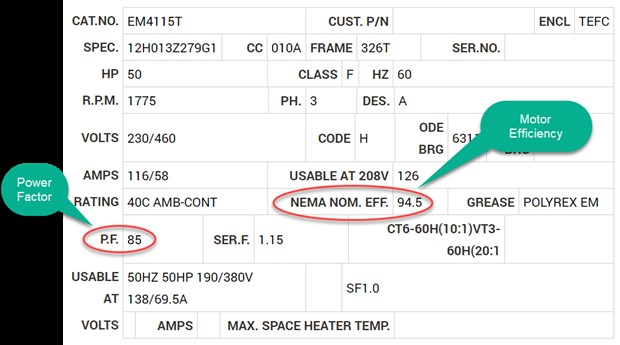It is relatively easy to calculate how much horsepower a fan is consuming in the field. Once calculated, this information can be used to identify the additional amount of flow and pressure the fan can produce within the limitations of the motor. To perform the calculations, we just need two field measurements which include the motors amperage draw and the line voltage (both can be measured with a voltage meter) and two items shown on the motor’s nameplate. This includes motor efficiency (often shown as “NEMA NOM. EFF.”) and power factor (shown as “PF”).

The formula used to calculate motor horsepower is straight forward:

Horsepower (3 phase) = (Volts x Amps x Efficiency x 1.732 x Power Factor) ÷ 746

If the above 50 hp motor is operating at 466 volts and drawing 43 amps (average across each of the 3 electrical leads), the fan’s operating horsepower can be calculated:

(466 Volts x 43 Amps x 1.732 x  0.945 Efficiency x 0.85 Power Factor) ÷ 746 = 37.37 Horsepower

Note that this result includes the fan’s drive losses (bearings and v-belt drive/or coupling). Using the following  variation of the fan laws, this information can be helpful in determining how much the fan’s speed can be increased within the limitations of the electrical systems and how much additional flow the fan can produce.

RPMnew = 3√(Horespowernew ÷ Horespowerold) x RPMold

CFMnew = RPMnew ÷ RPMold

If the existing fan is currently moving 20,000 CFM, generating 10” SP, consuming 37.37 horsepower, operating at 1300 rpm and has a maximum operating speed of 1500 rpm, how much additional flow can it produce if the 50 horsepower motor is maxed out:

3√(50 Horsepowernew ÷ 37.37 Horespowerold) x 1,300 RPMold = 1,432 RPMnew

Since the speed is less than 1500 RPM, we can calculate out the new flow and pressure:

1,432 RPMnew ÷ 1,300 RPMold = 22,031 CFMnew

(1,432 RPMnew ÷ 1,300 RPMold)2 x 10” SP = 12” SP